# Stats#

## Utilities#

### correlation()#

cor(x, y, method='pearson', show=False)[source]#

Density estimation

Computes kernel density estimates.

Parameters
• x (Union[list, np.array, pd.Series]) – Vectors of values.

• y (Union[list, np.array, pd.Series]) – Vectors of values.

• method (str) – Correlation method. Can be one of `"pearson"`, `"spearman"`, `"kendall"`.

• show (bool) – Draw a scatterplot with a regression line.

Returns

r – The correlation coefficient.

Examples

```In : import neurokit2 as nk

In : x = [1, 2, 3, 4, 5]

In : y = [3, 1, 5, 6, 6]

In : corr = nk.cor(x, y, method="pearson", show=True)

In : corr
Out: 0.80225745323842
```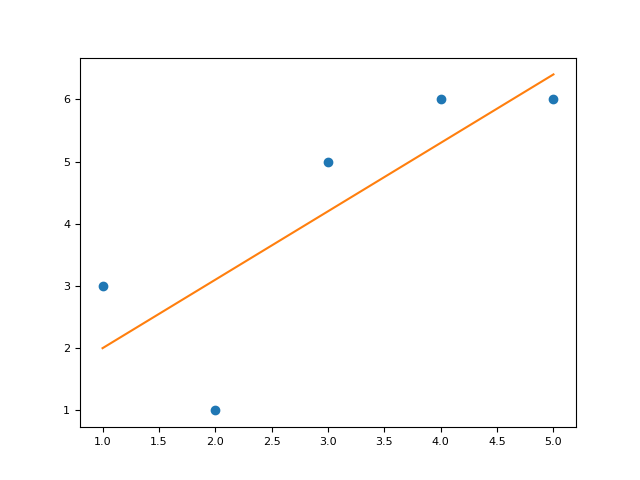### density()#

density(x, desired_length=100, bandwidth='Scott', show=False, **kwargs)[source]#

Density estimation.

Computes kernel density estimates.

Parameters
• x (Union[list, np.array, pd.Series]) – A vector of values.

• desired_length (int) – The amount of values in the returned density estimation.

• bandwidth (float) – Passed to the `method` argument from the `density_bandwidth()` function.

• show (bool) – Display the density plot.

• **kwargs – Additional arguments to be passed to `density_bandwidth()`.

Returns

• x – The x axis of the density estimation.

• y – The y axis of the density estimation.

`density_bandwidth`

Examples

```In : import neurokit2 as nk

In : signal = nk.ecg_simulate(duration=20)

In : x, y = nk.density(signal, bandwidth=0.5, show=True)
```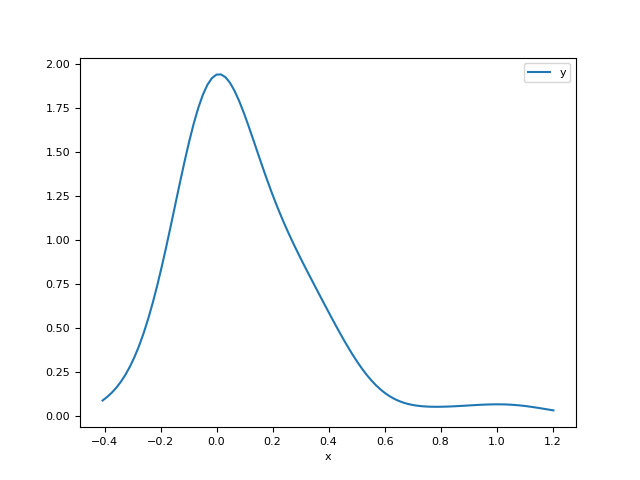```# Bandwidth comparison
In : _, y2 = nk.density(signal, bandwidth=1)

In : _, y3 = nk.density(signal, bandwidth=2)

In : _, y4 = nk.density(signal, bandwidth="scott")

In : _, y5 = nk.density(signal, bandwidth="silverman")

In : _, y6 = nk.density(signal, bandwidth="kernsmooth")

In : nk.signal_plot([y, y2, y3, y4, y5, y6],
...:                labels=["0.5", "1", "2", "Scott", "Silverman", "KernSmooth"])
...:
```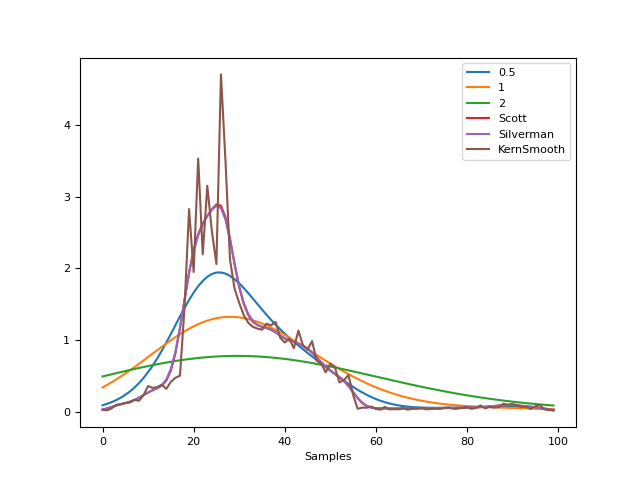### distance()#

distance(X=None, method='mahalanobis')[source]#

Distance

Compute distance using different metrics.

Parameters
• X (array or DataFrame) – A dataframe of values.

• method (str) – The method to use. One of `"mahalanobis"` or `"mean"` for the average distance from the mean.

Returns

array – Vector containing the distance values.

Examples

```In : import neurokit2 as nk

In : data = nk.data("iris").drop("Species", axis=1)

In : data["Distance"] = nk.distance(data, method="mahalanobis")

In : fig = data.plot(x="Petal.Length", y="Petal.Width", s="Distance", c="Distance", kind="scatter")
```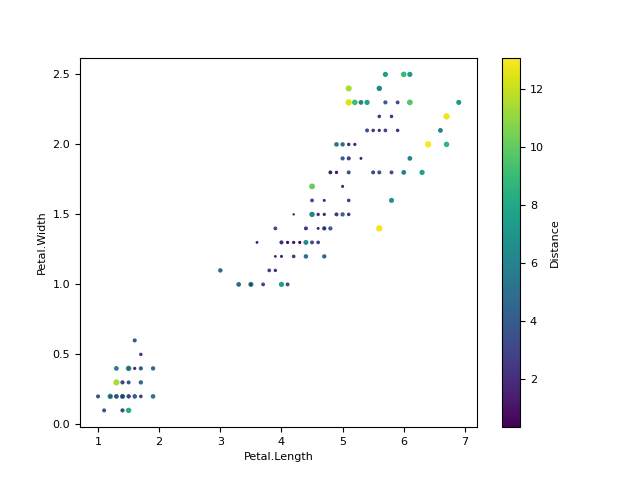```In : data["DistanceZ"] = np.abs(nk.distance(data.drop("Distance", axis=1), method="mean"))

In : fig = data.plot(x="Petal.Length", y="Sepal.Length", s="DistanceZ", c="DistanceZ", kind="scatter")
```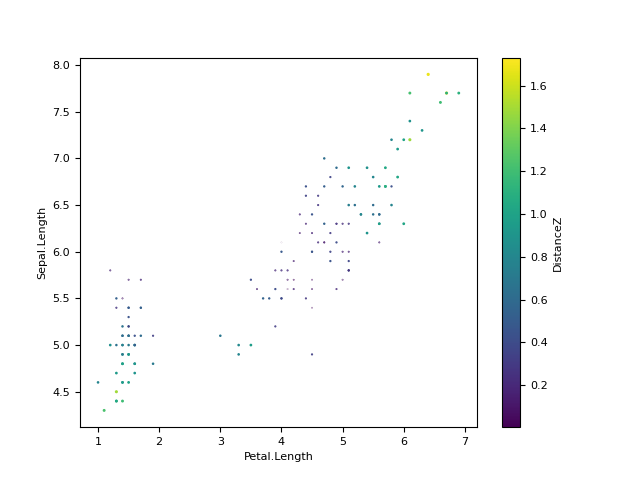### hdi()#

hdi(x, ci=0.95, show=False, **kwargs)[source]#

Highest Density Interval (HDI)

Compute the Highest Density Interval (HDI) of a distribution. All points within this interval have a higher probability density than points outside the interval. The HDI can be used in the context of uncertainty characterisation of posterior distributions (in the Bayesian farmework) as Credible Interval (CI). Unlike equal-tailed intervals that typically exclude 2.5% from each tail of the distribution and always include the median, the HDI is not equal-tailed and therefore always includes the mode(s) of posterior distributions.

Parameters
• x (Union[list, np.array, pd.Series]) – A vector of values.

• ci (float) – Value of probability of the (credible) interval - CI (between 0 and 1) to be estimated. Default to .95 (95%).

• show (bool) – If `True`, the function will produce a figure.

• **kwargs (Line2D properties) – Other arguments to be passed to `nk.density()`.

Returns

• float(s) – The HDI low and high limits.

• fig – Distribution plot.

Examples

```In : import numpy as np

In : import neurokit2 as nk

In : x = np.random.normal(loc=0, scale=1, size=100000)

In : ci_min, ci_high = nk.hdi(x, ci=0.95, show=True)
```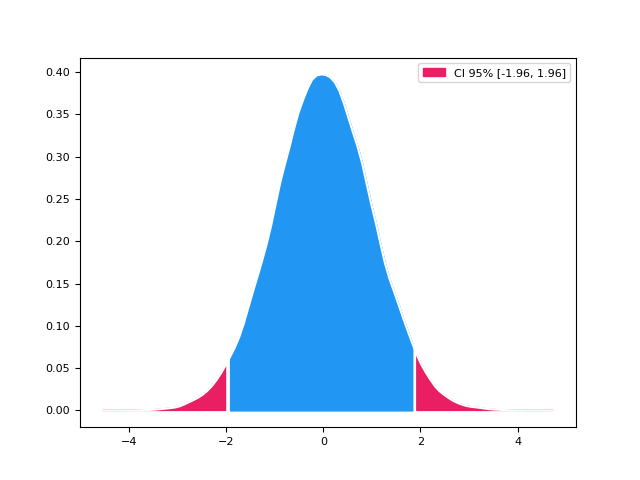Median Absolute Deviation: a “robust” version of standard deviation

Parameters
• x (Union[list, np.array, pd.Series]) – A vector of values.

• constant (float) – Scale factor. Use 1.4826 for results similar to default R.

Returns

Examples

```In : import neurokit2 as nk

In : nk.mad([2, 8, 7, 5, 4, 12, 5, 1])
Out: 3.7064999999999997
```

References

### rescale()#

rescale(data, to=[0, 1], scale=None)[source]#

Rescale data

Rescale a numeric variable to a new range.

Parameters
• data (Union[list, np.array, pd.Series]) – Raw data.

• to (list) – New range of values of the data after rescaling.

• scale (list) – A list or tuple of two values specifying the actual range of the data. If `None`, the minimum and the maximum of the provided data will be used.

Returns

list – The rescaled values.

Examples

```In : import neurokit2 as nk

In : nk.rescale([3, 1, 2, 4, 6], to=[0, 1])
Out: [0.4, 0.0, 0.2, 0.6000000000000001, 1.0]
```

### standardize()#

standardize(data, robust=False, window=None, **kwargs)[source]#

Standardization of data

Performs a standardization of data (Z-scoring), i.e., centering and scaling, so that the data is expressed in terms of standard deviation (i.e., mean = 0, SD = 1) or Median Absolute Deviance (median = 0, MAD = 1).

Parameters
• data (Union[list, np.array, pd.Series]) – Raw data.

• robust (bool) – If `True`, centering is done by substracting the median from the variables and dividing it by the median absolute deviation (MAD). If `False`, variables are standardized by substracting the mean and dividing it by the standard deviation (SD).

• window (int) – Perform a rolling window standardization, i.e., apply a standardization on a window of the specified number of samples that rolls along the main axis of the signal. Can be used for complex detrending.

• **kwargs (optional) – Other arguments to be passed to `pandas.rolling()`.

Returns

list – The standardized values.

Examples

```In : import neurokit2 as nk

In : import pandas as pd

# Simple example
In : nk.standardize([3, 1, 2, 4, 6, np.nan])
Out:
[-0.10397504898200735,
-1.14372553880208,
-0.6238502938920437,
0.41590019592802896,
1.4556506857481015,
nan]

In : nk.standardize([3, 1, 2, 4, 6, np.nan], robust=True)
Out:
[0.0,
-1.3489815189531904,
-0.6744907594765952,
0.6744907594765952,
2.023472278429786,
nan]

In : nk.standardize(np.array([[1, 2, 3, 4], [5, 6, 7, 8]]).T)
Out:
array([[-1.161895  , -1.161895  ],
[-0.38729833, -0.38729833],
[ 0.38729833,  0.38729833],
[ 1.161895  ,  1.161895  ]])

In : nk.standardize(pd.DataFrame({"A": [3, 1, 2, 4, 6, np.nan],
...:                              "B": [3, 1, 2, 4, 6, 5]}))
...:
Out:
A         B
0 -0.103975 -0.267261
1 -1.143726 -1.336306
2 -0.623850 -0.801784
3  0.415900  0.267261
4  1.455651  1.336306
5       NaN  0.801784

# Rolling standardization of a signal
In : signal = nk.signal_simulate(frequency=[0.1, 2], sampling_rate=200)

In : z = nk.standardize(signal, window=200)

In : nk.signal_plot([signal, z], standardize=True)
```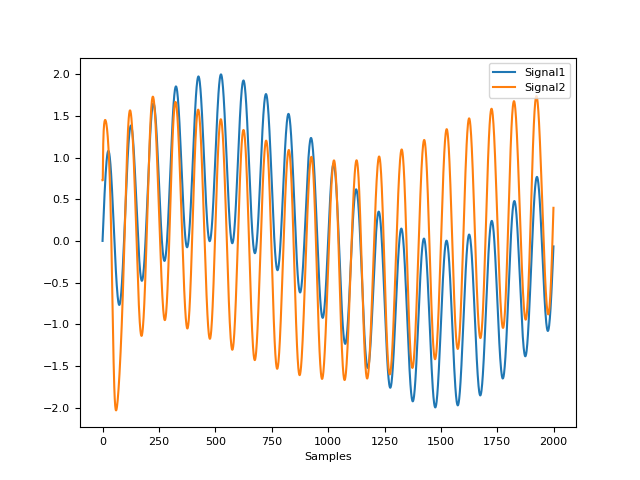### summary()#

summary_plot(x, errorbars=0, **kwargs)[source]#

Descriptive plot

Visualize a distribution with density, histogram, boxplot and rugs plots all at once.

Examples

```In : import neurokit2 as nk

In : import numpy as np

In : x = np.random.normal(size=100)

In : fig = nk.summary_plot(x)
```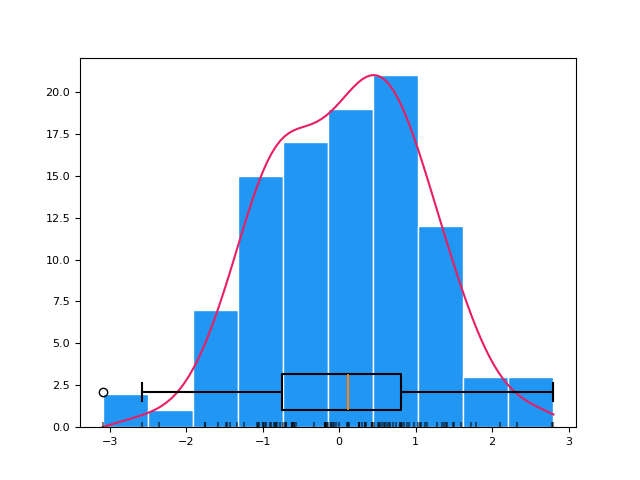## Clustering#

### cluster()#

cluster(data, method='kmeans', n_clusters=2, random_state=None, optimize=False, **kwargs)[source]#

Data Clustering

Performs clustering of data using different algorithms.

• kmod: Modified k-means algorithm.

• kmeans: Normal k-means.

• kmedoids: k-medoids clustering, a more stable version of k-means.

• pca: Principal Component Analysis.

• ica: Independent Component Analysis.

• aahc: Atomize and Agglomerate Hierarchical Clustering. Computationally heavy.

• hierarchical

• spectral

• mixture

• mixturebayesian

See `sklearn` for methods details.

Parameters
• data (np.ndarray) – Matrix array of data (E.g., an array (channels, times) of M/EEG data).

• method (str) – The algorithm for clustering. Can be one of `"kmeans"` (default), `"kmod"`, `"kmedoids"`, `"pca"`, `"ica"`, `"aahc"`, `"hierarchical"`, `"spectral"`, `"mixture"`, `"mixturebayesian"`.

• n_clusters (int) – The desired number of clusters.

• random_state (Union[int, numpy.random.RandomState]) – The `RandomState` for the random number generator. Defaults to `None`, in which case a different random state is chosen each time this function is called.

• optimize (bool) – Optimized method in Poulsen et al. (2018) for the k-means modified method.

• **kwargs – Other arguments to be passed into `sklearn` functions.

Returns

• clustering (DataFrame) – Information about the distance of samples from their respective clusters.

• clusters (np.ndarray) – Coordinates of cluster centers, which has a shape of n_clusters x n_features.

• info (dict) – Information about the number of clusters, the function and model used for clustering.

Examples

```In : import neurokit2 as nk

In : import matplotlib.pyplot as plt

In : data = nk.data("iris").drop("Species", axis=1)

# Cluster using different methods
In : clustering_kmeans, clusters_kmeans, info = nk.cluster(data, method="kmeans", n_clusters=3)

In : clustering_spectral, clusters_spectral, info = nk.cluster(data, method="spectral", n_clusters=3)

In : clustering_hierarchical, clusters_hierarchical, info = nk.cluster(data, method="hierarchical", n_clusters=3)

In : clustering_agglomerative, clusters_agglomerative, info= nk.cluster(data, method="agglomerative", n_clusters=3)

In : clustering_mixture, clusters_mixture, info = nk.cluster(data, method="mixture", n_clusters=3)

In : clustering_bayes, clusters_bayes, info = nk.cluster(data, method="mixturebayesian", n_clusters=3)

In : clustering_pca, clusters_pca, info = nk.cluster(data, method="pca", n_clusters=3)

In : clustering_ica, clusters_ica, info = nk.cluster(data, method="ica", n_clusters=3)

In : clustering_kmod, clusters_kmod, info = nk.cluster(data, method="kmod", n_clusters=3)

In : clustering_kmedoids, clusters_kmedoids, info = nk.cluster(data, method="kmedoids", n_clusters=3)

In : clustering_aahc, clusters_aahc, info = nk.cluster(data, method='aahc_frederic', n_clusters=3)

# Visualize classification and 'average cluster'
In : fig, axes = plt.subplots(ncols=2, nrows=5)

In : axes[0, 0].scatter(data.iloc[:,], data.iloc[:,], c=clustering_kmeans['Cluster'])
Out: <matplotlib.collections.PathCollection at 0x7f3826f9d600>

In : axes[0, 0].scatter(clusters_kmeans[:, 2], clusters_kmeans[:, 3], c='red')
Out: <matplotlib.collections.PathCollection at 0x7f3826f9d7e0>

In : axes[0, 0].set_title("k-means")
Out: Text(0.5, 1.0, 'k-means')

In : axes[0, 1].scatter(data.iloc[:,], data.iloc[:, ], c=clustering_spectral['Cluster'])
Out: <matplotlib.collections.PathCollection at 0x7f382c6dd5a0>

In : axes[0, 1].scatter(clusters_spectral[:, 2], clusters_spectral[:, 3], c='red')
Out: <matplotlib.collections.PathCollection at 0x7f38541ccc10>

In : axes[0, 1].set_title("Spectral")
Out: Text(0.5, 1.0, 'Spectral')

In : axes[1, 0].scatter(data.iloc[:,], data.iloc[:,], c=clustering_hierarchical['Cluster'])
Out: <matplotlib.collections.PathCollection at 0x7f3826f9f340>

In : axes[1, 0].scatter(clusters_hierarchical[:, 2], clusters_hierarchical[:, 3], c='red')
Out: <matplotlib.collections.PathCollection at 0x7f3825b562f0>

In : axes[1, 0].set_title("Hierarchical")
Out: Text(0.5, 1.0, 'Hierarchical')

In : axes[1, 1].scatter(data.iloc[:,], data.iloc[:,], c=clustering_agglomerative['Cluster'])
Out: <matplotlib.collections.PathCollection at 0x7f3823771900>

In : axes[1, 1].scatter(clusters_agglomerative[:, 2], clusters_agglomerative[:, 3], c='red')
Out: <matplotlib.collections.PathCollection at 0x7f38541cefb0>

In : axes[1, 1].set_title("Agglomerative")
Out: Text(0.5, 1.0, 'Agglomerative')

In : axes[2, 0].scatter(data.iloc[:,], data.iloc[:,], c=clustering_mixture['Cluster'])
Out: <matplotlib.collections.PathCollection at 0x7f382c6de770>

In : axes[2, 0].scatter(clusters_mixture[:, 2], clusters_mixture[:, 3], c='red')
Out: <matplotlib.collections.PathCollection at 0x7f382c6de710>

In : axes[2, 0].set_title("Mixture")
Out: Text(0.5, 1.0, 'Mixture')

In : axes[2, 1].scatter(data.iloc[:,], data.iloc[:,], c=clustering_bayes['Cluster'])
Out: <matplotlib.collections.PathCollection at 0x7f3828f3e890>

In : axes[2, 1].scatter(clusters_bayes[:, 2], clusters_bayes[:, 3], c='red')
Out: <matplotlib.collections.PathCollection at 0x7f382c6dc100>

In : axes[2, 1].set_title("Bayesian Mixture")
Out: Text(0.5, 1.0, 'Bayesian Mixture')

In : axes[3, 0].scatter(data.iloc[:,], data.iloc[:,], c=clustering_pca['Cluster'])
Out: <matplotlib.collections.PathCollection at 0x7f38179dec20>

In : axes[3, 0].scatter(clusters_pca[:, 2], clusters_pca[:, 3], c='red')
Out: <matplotlib.collections.PathCollection at 0x7f382c6de5c0>

In : axes[3, 0].set_title("PCA")
Out: Text(0.5, 1.0, 'PCA')

In : axes[3, 1].scatter(data.iloc[:,], data.iloc[:,], c=clustering_ica['Cluster'])
Out: <matplotlib.collections.PathCollection at 0x7f38179dc6d0>

In : axes[3, 1].scatter(clusters_ica[:, 2], clusters_ica[:, 3], c='red')
Out: <matplotlib.collections.PathCollection at 0x7f38179deef0>

In : axes[3, 1].set_title("ICA")
Out: Text(0.5, 1.0, 'ICA')

In : axes[4, 0].scatter(data.iloc[:,], data.iloc[:,], c=clustering_kmod['Cluster'])
Out: <matplotlib.collections.PathCollection at 0x7f38179dc460>

In : axes[4, 0].scatter(clusters_kmod[:, 2], clusters_kmod[:, 3], c='red')
Out: <matplotlib.collections.PathCollection at 0x7f38179ddd50>

In : axes[4, 0].set_title("modified K-means")
Out: Text(0.5, 1.0, 'modified K-means')

In : axes[4, 1].scatter(data.iloc[:,], data.iloc[:,], c=clustering_aahc['Cluster'])
Out: <matplotlib.collections.PathCollection at 0x7f382b0f8b80>

In : axes[4, 1].scatter(clusters_aahc[:, 2], clusters_aahc[:, 3], c='red')
Out: <matplotlib.collections.PathCollection at 0x7f38179deda0>

In : axes[4, 1].set_title("AAHC (Frederic's method)")
Out: Text(0.5, 1.0, "AAHC (Frederic's method)")
```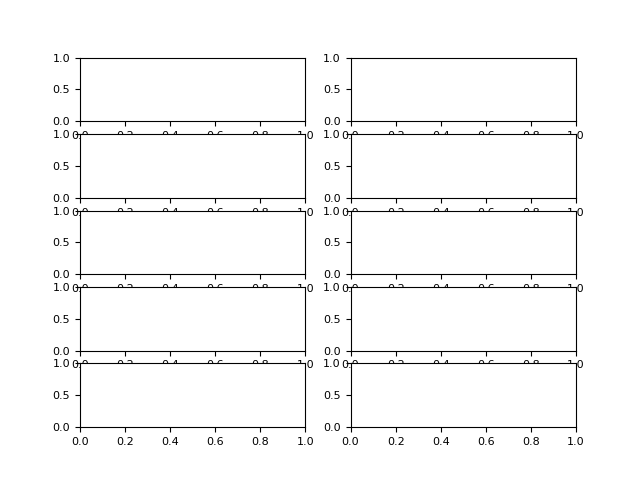References

• Park, H. S., & Jun, C. H. (2009). A simple and fast algorithm for K-medoids clustering. Expert systems with applications, 36(2), 3336-3341.

### cluster_findnumber()#

cluster_findnumber(data, method='kmeans', n_max=10, show=False, **kwargs)[source]#

Optimal Number of Clusters

Find the optimal number of clusters based on different indices of quality of fit.

Parameters
• data (np.ndarray) – An array (channels, times) of M/EEG data.

• method (str) – The clustering algorithm to be passed into `nk.cluster()`.

• n_max (int) – Runs the clustering alogrithm from 1 to n_max desired clusters in `nk.cluster()` with quality metrices produced for each cluster number.

• show (bool) – Plot indices normalized on the same scale.

• **kwargs – Other arguments to be passed into `nk.cluster()` and `nk.cluster_quality()`.

Returns

DataFrame – The different quality scores for each number of clusters:

• Score_Silhouette

• Score_Calinski

• Score_Bouldin

• Score_VarianceExplained

• Score_GAP

• Score_GAPmod

• Score_GAP_diff

• Score_GAPmod_diff

Examples

```In : import neurokit2 as nk

In : data = nk.data("iris").drop("Species", axis=1)

# How many clusters
In : results = nk.cluster_findnumber(data, method="kmeans", show=True)
```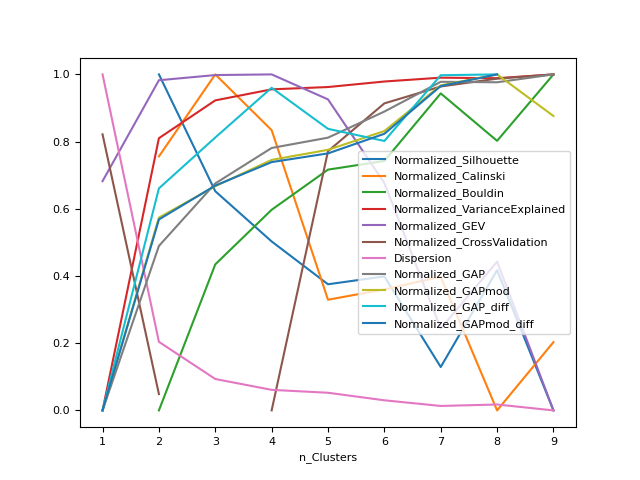### cluster_quality()#

cluster_quality(data, clustering, clusters=None, info=None, n_random=10, **kwargs)[source]#

Assess Clustering Quality

Compute quality of the clustering using several metrics.

Parameters
• data (np.ndarray) – A matrix array of data (e.g., channels, sample points of M/EEG data)

• clustering (DataFrame) – Information about the distance of samples from their respective clusters, generated from `cluster()`.

• clusters (np.ndarray) – Coordinates of cluster centers, which has a shape of n_clusters x n_features, generated from `cluster()`.

• info (dict) – Information about the number of clusters, the function and model used for clustering, generated from `cluster()`.

• n_random (int) – The number of random initializations to cluster random data for calculating the GAP statistic.

• **kwargs – Other argument to be passed on, for instance `GFP` as `'sd'` in microstates.

Returns

• individual (DataFrame) – Indices of cluster quality scores for each sample point.

• general (DataFrame) – Indices of cluster quality scores for all clusters.

Examples

```In : import neurokit2 as nk

In : data = nk.data("iris").drop("Species", axis=1)

# Cluster
In : clustering, clusters, info = nk.cluster(data, method="kmeans", n_clusters=3)

# Compute indices of clustering quality
In : individual, general = nk.cluster_quality(data, clustering, clusters, info)

In : general
Out:
n_Clusters  Score_Silhouette  ...  Score_GAP_sk  Score_GAPmod_sk
0         3.0          0.552819  ...      0.322841      1102.118786

[1 rows x 12 columns]
```

References

• Tibshirani, R., Walther, G., & Hastie, T. (2001). Estimating the number of clusters in a data set via the gap statistic. Journal of the Royal Statistical Society: Series B (Statistical Methodology), 63(2), 411-423.

• Mohajer, M., Englmeier, K. H., & Schmid, V. J. (2011). A comparison of Gap statistic definitions with and without logarithm function. arXiv preprint arXiv:1103.4767.

## Indices of fit#

### fit_error()#

fit_error(y, y_predicted, n_parameters=2)[source]#

Calculate the fit error for a model

Also specific and direct access functions can be used, such as `fit_mse()`, `fit_rmse()` and `fit_r2()`.

Parameters
• y (Union[list, np.array, pd.Series]) – The response variable (the y axis).

• y_predicted (Union[list, np.array, pd.Series]) – The fitted data generated by a model.

• n_parameters (int) – Number of model parameters (for the degrees of freedom used in R2).

Returns

dict – A dictionary containing different indices of fit error.

`fit_mse`, `fit_rmse`, `fit_r2`

Examples

```In : import neurokit2 as nk

In : y = np.array([-1.0, -0.5, 0, 0.5, 1])

In : y_predicted = np.array([0.0, 0, 0, 0, 0])

# Master function
In : x = nk.fit_error(y, y_predicted)

In : x
Out:
{'SSE': 2.5,
'MSE': 0.5,
'RMSE': 0.7071067811865476,
'R2': 0.7071067811865475,

# Direct access
In : nk.fit_mse(y, y_predicted)
Out: 0.5

In : nk.fit_rmse(y, y_predicted)
Out: 0.7071067811865476

Out: 0.7071067811865475

In : nk.fit_r2(y, y_predicted, adjusted=True, n_parameters=2)
Out: 0.057190958417936755
```

### fit_loess()#

fit_loess(y, X=None, alpha=0.75, order=2)[source]#

Local Polynomial Regression (LOESS)

Performs a LOWESS (LOcally WEighted Scatter-plot Smoother) regression.

Parameters
• y (Union[list, np.array, pd.Series]) – The response variable (the y axis).

• X (Union[list, np.array, pd.Series]) – Explanatory variable (the x axis). If `None`, will treat y as a continuous signal (useful for smoothing).

• alpha (float) – The parameter which controls the degree of smoothing, which corresponds to the proportion of the samples to include in local regression.

• order (int) – Degree of the polynomial to fit. Can be 1 or 2 (default).

Returns

• array – Prediction of the LOESS algorithm.

• dict – Dictionary containing additional information such as the parameters (`order` and `alpha`).

Examples

```In : import pandas as pd

In : import neurokit2 as nk

# Simulate Signal
In : signal = np.cos(np.linspace(start=0, stop=10, num=1000))

In : distorted = nk.signal_distort(signal,
...:                               noise_amplitude=[0.3, 0.2, 0.1],
...:                               noise_frequency=[5, 10, 50])
...:

# Smooth signal using local regression
In : pd.DataFrame({ "Raw": distorted, "Loess_1": nk.fit_loess(distorted, order=1),
...:                "Loess_2": nk.fit_loess(distorted, order=2)}).plot()
...:
Out: <AxesSubplot:>
```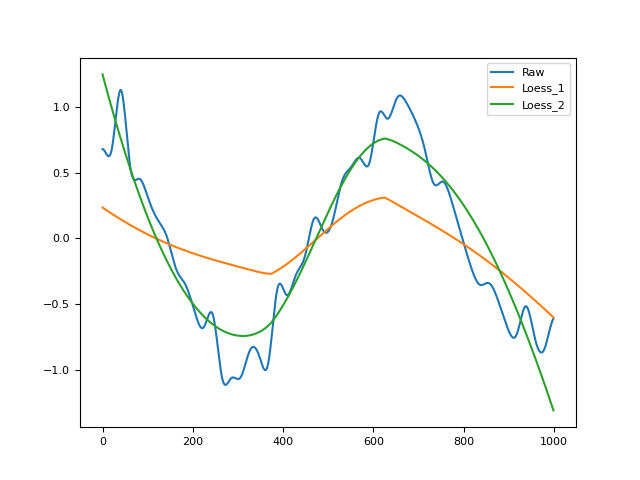References

### fit_mixture()#

fit_mixture(X=None, n_clusters=2)[source]#

Gaussian Mixture Model

Performs a polynomial regression of given order.

Parameters
• X (Union[list, np.array, pd.Series]) – The values to classify.

• n_clusters (int) – Number of components to look for.

Returns

• pd.DataFrame – DataFrame containing the probability of belonging to each cluster.

• dict – Dictionary containing additional information such as the parameters (`n_clusters()`).

Examples

```In : import pandas as pd

In : import neurokit2 as nk

In : x = nk.signal_simulate()

In : probs, info = nk.fit_mixture(x, n_clusters=2)  # Rmb to merge with main to return ``info``

In : fig = nk.signal_plot([x, probs["Cluster_0"], probs["Cluster_1"]], standardize=True)
```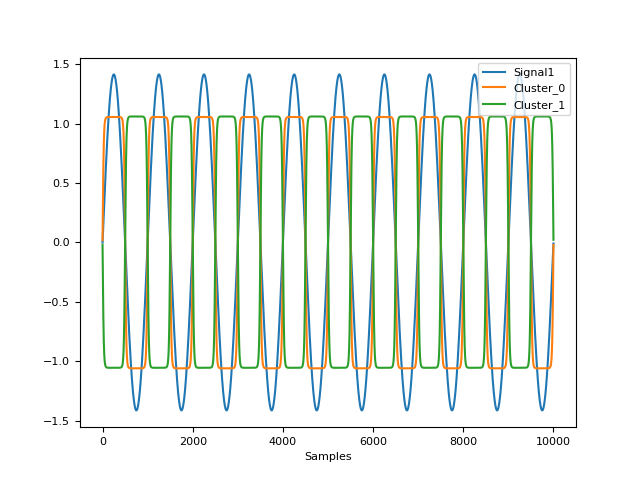### fit_polynomial()#

fit_polynomial(y, X=None, order=2, method='raw')[source]#

Polynomial Regression

Performs a polynomial regression of given order.

Parameters
• y (Union[list, np.array, pd.Series]) – The response variable (the y axis).

• X (Union[list, np.array, pd.Series]) – Explanatory variable (the x axis). If `None`, will treat y as a continuous signal.

• order (int) – The order of the polynomial. 0, 1 or > 1 for a baseline, linear or polynomial fit, respectively. Can also be `"auto"`, in which case it will attempt to find the optimal order to minimize the RMSE.

• method (str) – If `"raw"` (default), compute standard polynomial coefficients. If `"orthogonal"`, compute orthogonal polynomials (and is equivalent to R’s `poly` default behavior).

Returns

• array – Prediction of the regression.

• dict – Dictionary containing additional information such as the parameters (`order`) used and the coefficients (`coefs`).

`signal_detrend`, `fit_error`, `fit_polynomial_findorder`

Examples

```In : import pandas as pd

In : import neurokit2 as nk

In : y = np.cos(np.linspace(start=0, stop=10, num=100))

In : pd.DataFrame({"y": y,
...:               "Poly_0": nk.fit_polynomial(y, order=0),
...:               "Poly_1": nk.fit_polynomial(y, order=1),
...:               "Poly_2": nk.fit_polynomial(y, order=2),
...:               "Poly_3": nk.fit_polynomial(y, order=3),
...:               "Poly_5": nk.fit_polynomial(y, order=5),
...:               "Poly_auto": nk.fit_polynomial(y, order='auto')}).plot()
...:
Out: <AxesSubplot:>
```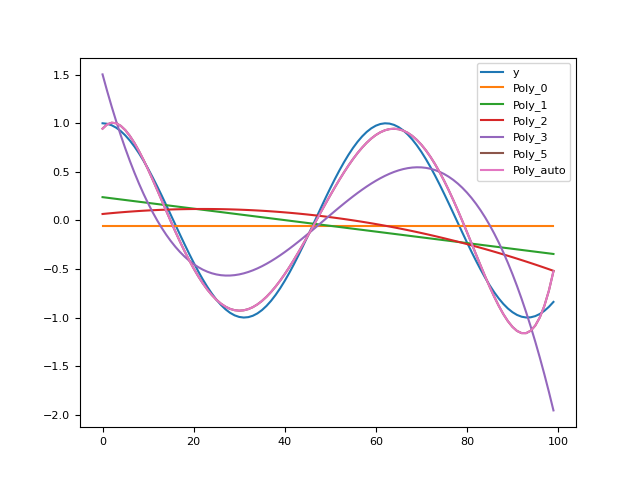Any function appearing below this point is not explicitly part of the documentation and should be added. Please open an issue if there is one.

Submodule for NeuroKit.

density_bandwidth(x, method='KernSmooth', resolution=401)[source]#

Bandwidth Selection for Density Estimation

Bandwidth selector for `density()` estimation. See `bw_method` argument in `scipy.stats.gaussian_kde()`.

The `"KernSmooth"` method is adapted from the `dpik()` function from the KernSmooth R package. In this case, it estimates the optimal AMISE bandwidth using the direct plug-in method with 2 levels for the Parzen-Rosenblatt estimator with Gaussian kernel.

Parameters
• x (Union[list, np.array, pd.Series]) – A vector of values.

• method (float) – The bandwidth of the kernel. The larger the values, the smoother the estimation. Can be a number, or `"scott"` or `"silverman"` (see `bw_method` argument in `scipy.stats.gaussian_kde()`), or `"KernSmooth"`.

• resolution (int) – Only when `method="KernSmooth"`. The number of equally-spaced points over which binning is performed to obtain kernel functional approximation (see `gridsize` argument in `KernSmooth::dpik()`).

Returns

float – Bandwidth value.

`density`

Examples

```In : import neurokit2 as nk

In : x = np.random.normal(0, 1, size=100)

In : bw = nk.density_bandwidth(x)

In : bw
Out: 0.3749973253781269

In : nk.density_bandwidth(x, method="scott")
Out: 0.3981071705534972

In : nk.density_bandwidth(x, method=1)
Out: 1

In : x, y = nk.density(signal, bandwidth=bw, show=True)
```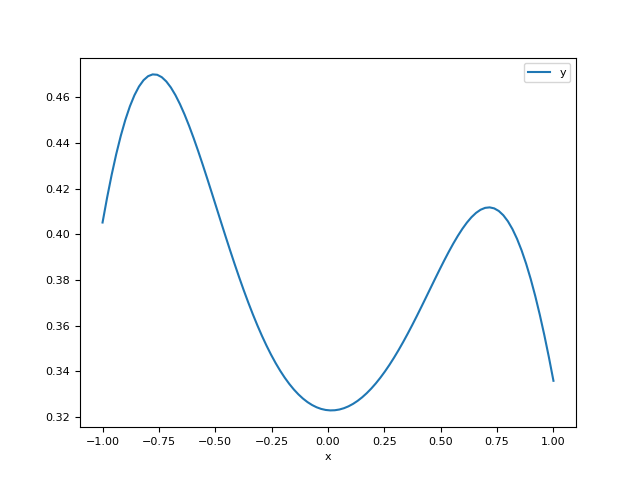References

• Jones, W. M. (1995). Kernel Smoothing, Chapman & Hall.

fit_mse(y, y_predicted)[source]#

Compute Mean Square Error (MSE).

fit_polynomial_findorder(y, X=None, max_order=6)[source]#

Polynomial Regression.

Find the optimal order for polynomial fitting. Currently, the only method implemented is RMSE minimization.

Parameters
• y (Union[list, np.array, pd.Series]) – The response variable (the y axis).

• X (Union[list, np.array, pd.Series]) – Explanatory variable (the x axis). If ‘None’, will treat y as a continuous signal.

• max_order (int) – The maximum order to test.

Returns

int – Optimal order.

`fit_polynomial`

Examples

import neurokit2 as nk

y = np.cos(np.linspace(start=0, stop=10, num=100))

nk.fit_polynomial_findorder(y, max_order=10)

9